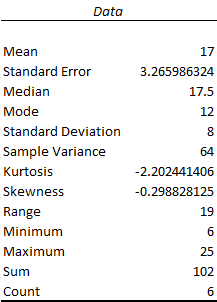# Consider a sample with six observations of 24, 25, 12, 6, 23, and 12. Compute the z-score for each observation.

Question
2 views

Consider a sample with six observations of 24, 25, 12, 6, 23, and 12. Compute the z-score for each observation.

check_circle

star
star
star
star
star
1 Rating
Step 1

Step-by-step procedure to obtain the mean and standard deviation using Excel.

• In Excel sheet, enter Data.
• In Data, select Data Analysis and choose Descriptive statistics.
• In Input Range, select Data.
• click Labels in First Row.
• Select Summary Statistics.
• Click Ok.

Output using Excel is given below:From the output, the mean is 17, and the standard deviation is 8.

...

### Want to see the full answer?

See Solution

#### Want to see this answer and more?

Solutions are written by subject experts who are available 24/7. Questions are typically answered within 1 hour.*

See Solution
*Response times may vary by subject and question.
Tagged in

### Statistics